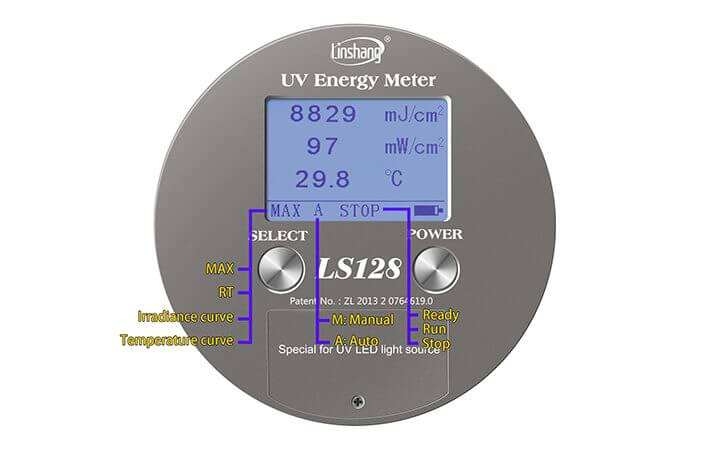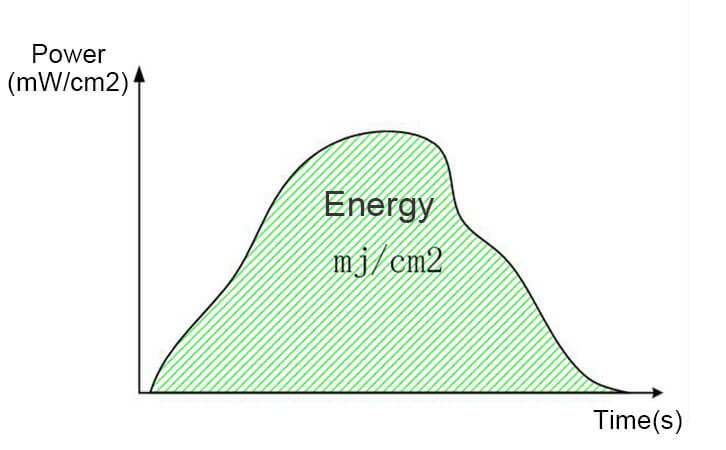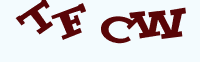# The Principle of Linshang UV Energy Meter and UV Integrating Radiometer

Time:2019/02/27 18:24:00 Browse:877

In the UV curing and exposure industry, the relevant process parameters need to be confirmed according to the UV energy value. We all know a physical equation: Energy =Power *Time, but this equation is established only when the power is constant. Typically, the power of the UV light source is impossible to remain stable.
The Linshang
UV integrating radiometercan automatically calculate the UV energy value, no manual calculation required.
In the UV curing and exposure industry, the energy value is one of the important indexes to confirm the process parameters, but what exactly does the energy value mean?
Customers often reflect that the power value measured with the UV energy meter multiplied by the time is not consistent with the UV energy value displayed by the UV energy meter.In fact, in the case of power stability unchanged, the energy value is equal to the power multiplied by the time, but in the actual application, the power value of the UV light source is impossible to stabilize unchanged. To give a simple example, the speed of a car cannot be constant in the course of driving, so multiplying the speed of a certain moment by time does not calculate the distance the car is travelling. The correct way to calculate the distance is to multiply the average speed of the car during this time by time. Similarly, the power value shown on the UV integrating radiometeris a real-time power value, so when calculating the energy value, we need to calculate the energy value through a large amount of data, that is, to integrate the power value over this period of time. As shown in the following figure, the horizontal coordinates are time, the longitudinal coordinates are power values, and you can see that the power values are irregularly variable. The area of the graph represents the energy value.The sampling speed of Linshang UV energy value can reach 2048 times per second, the high-speed sampling ensured accurate UV energy value. In addition to testing UV energy values and UV power values, UV integrating radiometercan also display temperature and power curves to help you better determine process parameters.

Enquiry

### EnquiryClick image refresh captcha
x